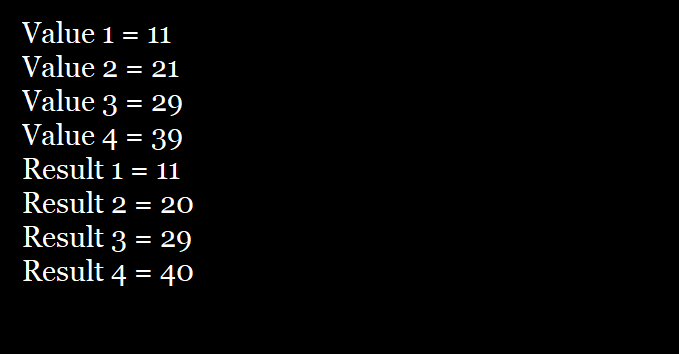# Java Operators Interview Questions

## 08 Nov Java Operators Interview Questions

Here’s Java Operators Interview Questions. The level of questions is suitable for beginners as well advanced core Java programmers. This part covers Java Operators, including Modulus operators, compound assignment operators, increment operators, etc.

### Q61. What is a Modulus Operator?

A61. Modulus operator is represented by % and returns the remainder of division operation.

### Q62. Show the usage of Modulus Operator with an example program.

A62. Here are two code snippets that returns the remainder using Modulus operator,

// Code snippet 1

Output

// Code snippet 2

Output

### Q63. What are Compound Assignment Operators?

A63. The operator that combines Arithmetic operator with assignment is known as Compound Assignment operator.

For example, x+=10;

### A63

. Here’s a simple example, with output,

Here’s the output,### Q64. Why compound assignment operators are used, since the same operation can be done normally as shown above?

A64. Compound assignment operators are used since they have a short form to do the same work, and they are more efficient that the longer form.

So, z+=25 is used more than z = z + 25 in coding.

### Q65. What is the difference between ++x and x++ under increment operators?

A65. In ++x, the value of x will get incremented before it is used in the expression.

In x++, the previous value is used in the expression first, and after that x is modified.

### Q66. Explain the above concept of increment operator with code snippets?

A66. 1.)
a = 10;
b = ++a;

In the above case, a is set to 11, since ++a increment before a is assigned to b.

2.)

a = 10;
b = a++;

In the above case, the value of a is assigned to be first before it gets increments by a++.

### Q67. Write a complete program explaining the increment and decrement operators in Java?

A67. Here’s the program,

Here’s the output,### Q68. What are Bitwise Logical Operators?

A68.  &, |, ^, and ~ are bitwise logical operators.

### Q69. What is the role of Bitwise NOT Operator (~)? Give an example?

A69. Bitwise Not Operator inverts all the bits of the operand.
For example, Bit pattern 1101 for the number 13

So, the value of ~1101 = 0010 i.e. all individual bits invert.

### Q70. What is Bitwise AND Operator (&). Give an example?

A70. The Bitwise AND operator fulfils the following two conditions,

• 1 bit if both operands are 1,
• 0 for all others

Here’s an example,

##### Tags:[email protected]

We work to create free tutorials for all.